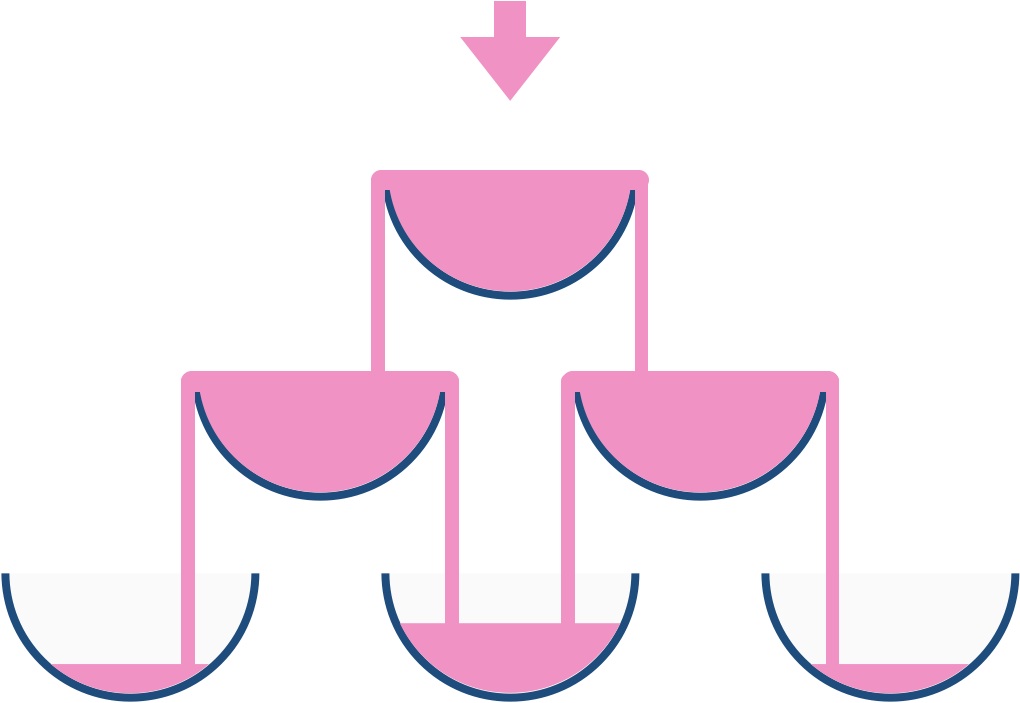# Water Overflow | 799. Champagne Tower Solution

GeeksforGeeks

There is a stack of water glasses in a form of pascal triangle and a person wants to pour the water at the topmost glass, but the capacity of each glass is 1 unit. Overflow takes place in such a way that after 1 unit, 1/2 of remaining unit gets into bottom left glass and other half in bottom right glass.Now John pours K units of water in the topmost glass and wants to know how much water is there in the Cth glass of the Rth row.

Note: Assume that there are enough glasses in the triangle till no glass overflows.

Example 1:

```Input:
K = 3, R = 2, C = 1
Output:
1.000000
Explanation:
After the first glass, 2 units of water
will remain and they will spread equally
on the two glasses on the second row.
Therefore, the glass on the 2nd row and
1st column will have 1 unit of water.```

Example 2:

```Input:
K = 3, R = 2, C = 2
Output:
1.000000
Explanation:
After the first glass, 2 units of water
will remain and they will spread equally
on the two glasses on the second row.
Therefore, the glass on the 2nd row and
2nd column will have 1 unit of water.```

You don't need to read input or print anything. Your task is to complete the function waterOverflow() which takes 3 Integers K, R and C as input and returns the amount of water(6 digits of precision) in the Cth glass of Rth row.

Expected Time Complexity: O(K2)
Expected Auxiliary Space: O(K2)

Constraints:
1 <= K <= 500
1 <= R,C <= K

LeetCode

We stack glasses in a pyramid, where the first row has `1` glass, the second row has `2` glasses, and so on until the 100th row.  Each glass holds one cup of champagne.

Then, some champagne is poured into the first glass at the top.  When the topmost glass is full, any excess liquid poured will fall equally to the glass immediately to the left and right of it.  When those glasses become full, any excess champagne will fall equally to the left and right of those glasses, and so on.  (A glass at the bottom row has its excess champagne fall on the floor.)

For example, after one cup of champagne is poured, the top most glass is full.  After two cups of champagne are poured, the two glasses on the second row are half full.  After three cups of champagne are poured, those two cups become full - there are 3 full glasses total now.  After four cups of champagne are poured, the third row has the middle glass half full, and the two outside glasses are a quarter full, as pictured below.Now after pouring some non-negative integer cups of champagne, return how full the `jth` glass in the `ith` row is (both `i` and `j` are 0-indexed.)

Example 1:

```Input: poured = 1, query_row = 1, query_glass = 1
Output: 0.00000
Explanation: We poured 1 cup of champange to the top glass of the tower (which is indexed as (0, 0)). There will be no excess liquid so all the glasses under the top glass will remain empty.
```

Example 2:

```Input: poured = 2, query_row = 1, query_glass = 1
Output: 0.50000
Explanation: We poured 2 cups of champange to the top glass of the tower (which is indexed as (0, 0)). There is one cup of excess liquid. The glass indexed as (1, 0) and the glass indexed as (1, 1) will share the excess liquid equally, and each will get half cup of champange.
```

Example 3:

```Input: poured = 100000009, query_row = 33, query_glass = 17
Output: 1.00000
```

Constraints:

• `0 <= poured <= 109`
• `0 <= query_glass <= query_row < 100`
Solution:

If we carefully read the question we can think about the pascal triangle.
We will pour the water to the top and if it overflows then it will start going to the glasses below it.
So we will pour everything into the top glass and then we will check whether it overflows or not if yes then we will add the extra to the two glasses below it. Let us get this through some images.

GFG

LeetCode